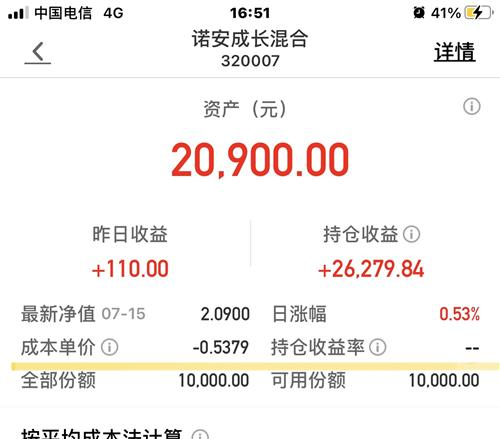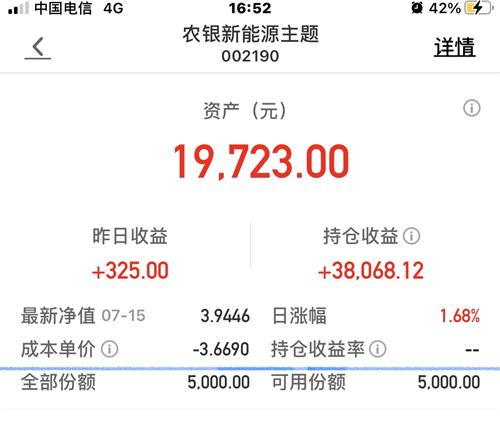1.让学生经历编制6的乘法口诀的过程，体验6的乘法口诀的来源，促使学生加深对每句口诀意义的理解，更好地掌握乘法口诀。
2.使学生熟记6的乘法口诀，能灵活运用6的乘法口诀解决问题。
3.培养学生认真观察、独立思考的良好习惯和推理概括能力，向学生渗透函数对应思想。
4.从学生的生活实际出发，激发学生学习数学的兴趣和参与的积极性，树立学生学好数学的信心，感受探索的乐趣。

4×3= 2×2= 1×3= 2×4= 2×2=

1
2
3
4
5
6

6
12

2、你能根据1×6=6，1×6=6编出一句乘法口诀吗？ （ 板书：一六得六）

（板书： 二六十二 三六十八 四六二十四 五六三十 六六三十六 ）

1）齐背 2）分组背 3）对口令 4）开火车背 5）指名背 6）同桌比赛，谁背得熟练

1、用口诀读下面的乘法算式
2×6= 3×6= 4×6= 6×2= 6×3= 6×4= 6×5= 4×5= 6×6= 1×6=
2、钓鱼小高手2×6= 4×6= 6×4= 1×6= 6×5= 6×6= 3×6= 6×2= 6×3= 5×6=
3、谜语： 有时挂在天上，有时挂在树梢。有时像个圆盘，有时像把镰刀。

（引导学生运用口诀解决问题）
4、根据图形说口诀和乘法算式

6的乘法口诀
1×6＝6 一六得六 6×1＝6
2×6＝12 二六十二 6×2＝12
3×6＝18 三六十八 6×3＝18
4×6＝24 四六二十四 6×4＝24
5×6＝30 五六三十 6×5＝30
6×6＝36 六六三十六

SGHL 1000万港币 基兆业、中华煤气 1000万港币 香港百仁基金 810万港币 香港南益集团国内总部 500万